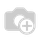# Asset Linear Depreciation Calculation On Non-Full(Partial) Month?

As I found, when the depreciation board of purchased Asset is computed, the first month linear deprecitaion is calculated in curious logic(When Prorata Temporis = "True"): Monthly Depreciatiion/Total Days in Year X (Days after Purchase Date Till End of Year). I beleive the correct logic is: Monthly Depr/Days in Purchase Month X (Days after purchase till end of month). These two will give totally different result. Is there any module to calculate with the second one? Can anyone explain why it calculates now with this method???

1 Commenthave you found an answer to this? Googling I found account_asset_management which might help, will post an update after testing it